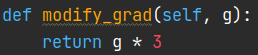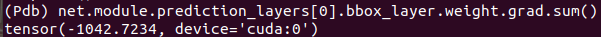# DistributedDataParallel modify gradient before averaging

Hi all! I think the “DistributedDataParallel” automatically average the gradient when calling “loss.backward()”. But is it possible to first compute the local gradient of the parameters, then do some modification to the local gradient, and finally average the gradient among the workers?

Thanks!

@anxu tensor.register_hook(customHook) may work for your case, you need to write customHook to modify grad of the tensor

1 Like

Hi Yanli,

I am not sure whether tensor.register_hook will work, but the documentation mentioned that,

Forward and backward hooks defined on `module` and its submodules won’t be invoked anymore, unless the hooks are initialized in the `forward()` method.

Besides I need to first collect the whole gradient and then do some modification. Now I am turning to torch.distributed.all_reduce, but it will be easier if there is a way to do this via DistributedDataParallel.

Hi, anxu @anxu , the “DistributedDataParallel” automatically average the gradient when calling “loss.backward()”,
But I didn’t find the corresponding script in pytorch source code, Do you know where it is ?

Hi, Yanli @Yanli_Zhao , the “DistributedDataParallel” automatically average the gradient when calling “loss.backward()”,
But I didn’t find the corresponding script in pytorch source code, Do you know where it is ?

DDP averages gradients by all-reducing them across participating processes (see https://pytorch.org/docs/stable/_modules/torch/distributed/distributed_c10d.html#all_reduce). Some specific bits that include gradient averaging can be found in the `allReduce` calls here: https://github.com/pytorch/pytorch/blob/master/torch/csrc/distributed/c10d/reducer.cpp

@Yanli_Zhao’s solution works great. You can register the hook either before or after DDP’ing the model. Though the docs say that hooks are removed, that’s either not actually the case or it doesn’t apply to hooks on the tensors themselves.

Here’s some demo code:

``````from torch.nn.parallel import DistributedDataParallel as DDP
from torch import nn
import torch
import os
import torch.distributed as dist
import torch.multiprocessing as mp

def setup(rank, world_size):
"""Setup code comes directly from the docs:

https://pytorch.org/tutorials/intermediate/ddp_tutorial.html
"""
os.environ['MASTER_PORT'] = '29500'

dist.init_process_group("nccl", rank=rank, world_size=world_size)
torch.manual_seed(42)

def cleanup():
dist.destroy_process_group()

def pre_average(g):
print(f'Pre-DDP hook ({g.device}): {g[0, 0]}')

def post_average(g):
print(f'Post-DDP hook ({g.device}): {g[0, 0]}')

def worker(rank, world_size):
# Set up multiprocessing stuff
setup(rank, world_size)

# Create a trivial model
model = nn.Linear(1, 1, bias=False).to(rank)
torch.nn.init.constant_(model.weight, 1.)

# Create some trivial data.
# Gradients for x = (1, 2) should be (2, 8)
x = torch.tensor([rank+1]).float().to(rank)

# Register a hook before and after DDP'ing the model
model.weight.register_hook(pre_average)
model = DDP(model, device_ids=[rank])
model.module.weight.register_hook(post_average)

# Backprop!
l = model(x).pow(2).sum()
l.backward()

# Check what's left in the gradient tensors

cleanup()

if  __name__ == '__main__':
world_size = 2
mp.spawn(worker,
args=(world_size,),
nprocs=world_size,
join=True)
``````

Run from the terminal, this should print

``````Pre-DDP hook  (cuda:0): 2.0
Post-DDP hook (cuda:0): 2.0
Pre-DDP hook  (cuda:1): 8.0
Post-DDP hook (cuda:1): 8.0
Final value   (cuda:0): 5.0
Final value   (cuda:1): 5.0
``````

Hi @anxu， I did some test. `Tensor.register_hook()` does work in DDP. I first wrap my model with DDP, then I modify the grad manually with a custom function in the `forward` code where I construct my model:Here’s the gradients after calling `loss.backward()`:You can see the gradients are just 3 times after being modified, which means `.register_hook()` works well in DDP.